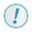Operator Types in Spatial Modeler

ERDAS IMAGINE Help

HGD_Variant
16.5.1
HGD_Product
ERDAS IMAGINE
HGD_Portfolio_Suite
Producer

These are the function types used in the Spatial Modeler (see below for details):

• Point
• Neighborhood
• Global
• Zonal
• Layer
• Point Cloud
• Combination

Point Operators

Point operators operate point by point. The standard rules for object type combinations generally apply to most point operators. Point operators usually operate on any object type. Point operators operating on a Raster consider the value of only one pixel in each input layer in determining the value for a single pixel of output. Point operators make up the majority of operators supported by the Modeler.

Max is an example of a point operator.

Each element of the output of Max is the maximum of the corresponding inputs.

Neighborhood Operators

Neighborhood operators operate on a raster using a neighborhood that is defined in a matrix. Neighborhood operators involve each pixel's neighbors, or nearby pixels, in the calculations.

Focal Max is an example of a neighborhood operator.

Each pixel in the output of Focal Max is the maximum of the neighbors of the corresponding pixel in the input Raster, using the Matrix Focus to define the size and shape of the neighborhood.

Global Operators

Global operators operate on an entire object or layer, and return a single value for that object or layer.

Statistics is an example of a global operator.

The computation of the statistics requires that all of the input be processed before the operator can make the results available.Models that include global operators may impact the performance of the preview.

Zonal Operators

Zonal operators operate on two input Raster layers, and return either a Matrix or Table. One of the two input layers is called the zone layer, and the values of this layer (which must be unsigned integer data type) are called zones. The second input layer is called the class layer or value layer. Statistics are computed from the class or value layer for each zone in the zone layer, and the results are returned in the output Table or Matrix. Each row of the returned object corresponds to one zone.

Summary is a Zonal operator that returns a Matrix. Zonal operators that return a Table include the versions of the Zonal Max, Zonal Min, Zonal Mean, Zonal Range, and Zonal Standard Deviation that have two Raster layers as input. The other versions of these operators use the output of Summary as input, and so are actually Point operators rather than Zonal operators, since their input is a Matrix, not two Raster layers.Models that include zonal operators may impact the performance of the preview.

Layer Operators

Layer operators operate on an entire Raster. Layer operators output a Raster. The value for each pixel in the output Raster may depend on the arrangement of pixel values over the entire input layer.

Layer operators include Search and Clump.Models that include layer operators may impact the performance of the preview.

Combination Operators

Some operators in the modeler are actually combinations of simpler operators.

An example is Principal Components, which combines Statistics, Eigenmatrix, Matrix Transpose and Linear Combination into one operator. Statistics is a global operator, while the others are point operators. Other combination operators include HistoEq, Stretch, and Raster Match.